Next: Tisserand criterion Up: Three-body problem Previous: Circular restricted three-body problem

# Jacobi integral

Consider the function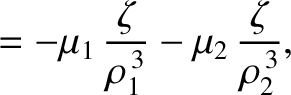(9.10)

The time derivative of this function is written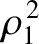(9.11)

Moreover, it follows, from Equations (9.3)-(9.4) and (9.8)-(9.9), that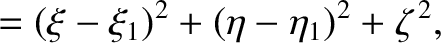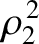(9.12) and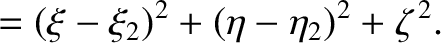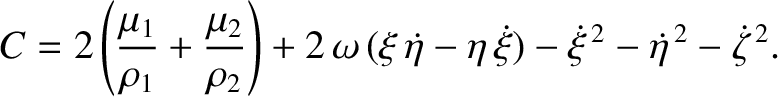(9.13)

Combining Equations (9.5)-(9.7) with the preceding three expressions, after considerable algebra (see Exercise 1), we obtain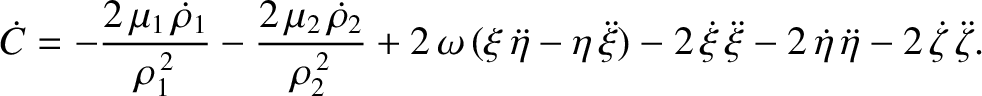(9.14)

In other words, the function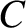--which is usually referred to as the Jacobi integral--is a constant of the motion.

We can rearrange Equation (9.10) to give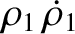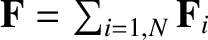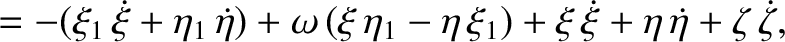(9.15)

where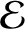is the energy (per unit mass) of mass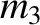,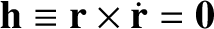the angular momentum (per unit mass) of mass, and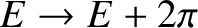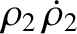the orbital angular velocity of the other two masses. Note, however, that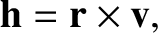is not a constant of the motion. Hence,is not a constant of the motion either. In fact, the Jacobi integral is the only constant of the motion in the circular restricted three-body problem. Incidentally, the energy of massis not a conserved quantity because the other two masses in the system are moving.Next: Tisserand criterion Up: Three-body problem Previous: Circular restricted three-body problem
Richard Fitzpatrick 2016-03-31